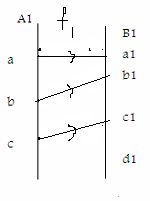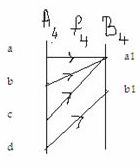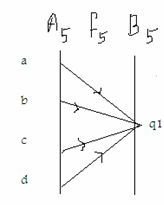Name: ___________________Date:___________________

 Email us to get an instant 20% discount on highly effective K-12 Math & English kwizNET Programs!

High School Mathematics - 24.7 Inverse of Functions

 Consider the function of of the set of children to the set of their mothers. It is obviously a many-one type function as several children may have the same mother. Its inverse however will not exist. In this case we will have a relation in which several ordered pairs of the form (mother, child) may have the same first component. Consider the functions f1, f2, f3, f4, f5 exhibited by the following diagrams:f1(one-one into type), f1-1 doesn't exist. f2(one-one onto type), f2-1 doesn't exist. f3(many-one into type), f3-1 doesn't exist. f4(many-one onto type), f4-1 doesn't exist. f5(constant type), f5-1 doesn't exist. The above illustrations lead to a conclusion that A function f: A -> B will have its inverse g: B -> A if and only if f is a one-one function. f is an onto function i.e f is bijective. Definition: If f is a function f: A - >B, then there will exist a function g: B ->A if f is a one-one onto function, and such that the range of f is the domain of g and domain of f is the range of g; then g is called the inverse of f and is denoted by f-1. Also f is called the inverse of g and is denoted as g-1. Symbolically, a function f: x - >y , then its inverse is represented as f-1: y - > x, or if y = f(x), then its inverse is represented as f-1(y) = x. Example: If f: R -> R be define by f(x) = x3 + 7, find a formula that defines f-1. Solution: Method to find the inverse of a bijective function Step 1: Put f(x) = y, where y € B and x €A. Step 2: Solve f(x) = y to obtainx in terms of y. Step 3: In the relation obtained in step 2, replace x by f-1 to obtain the inverse of f. Step 4: Let f(x) = x3 + 7 = y, then x3 = y - 7 Step 5: x = (y-7)1/3 = >f-1(y) = (y - 7)1/3 Answer: Hence f-1 : R -> R : f-1(x) = (x-7)1/3for all x € R. Directions: Solve the following problems. Also write at least 5 examples of your own.
 Q 1: Find the range of the function h(x) = x2 + 1{(y: y is real,y>=1}Range doesn't existRange = Domain Q 2: If f: R ->R be defined by f(x) = 3x- 4, then f-1(x) is (x+4)/3x/3 - 43x + 4 Q 3: If f: R=>R such that f(x) = e3x+2,find f-1e2105 Q 4: Let f : A -> B, find f-1(B).Inverse doesn't existBA Q 5: If f : R+ -> R such that f(x) = log8x,find f-1(x).log8y8x8y Q 6: If f: R ->R is such that f(x) = log3x, f-1 is equal to log x33x31/x Q 7: If f:R -> R, write the inverse of f(x) = 3x + y.(x-4)/3(y-4)/3-(3x+y) Q 8: If f(x) = (2x+1)/3, for each x € R, find f-1.(-2x-1)/3(3x-1)/2(2x-1)/3 Question 9: This question is available to subscribers only! Question 10: This question is available to subscribers only!